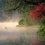# Doubt in calculus limit problem

Determine $\displaystyle{\lim_{n\to\infty} x_n}$ if $\left(1+\frac{1}{n}\right)^{n+x_n}=e$

I have typed 2 methods giving two different answers

## Method 1

$\lim_{n\to\infty}\left(1+\frac{1}{n}\right)^{n+x_n}=\lim_{n\to\infty}e\\\implies \lim_{n\to\infty}\left(1+\frac{1}{n}\right)^{n}\cdot\lim_{n\to\infty}\left(1+\frac{1}{n}\right)^{x_n}=e\\\implies e\cdot\lim_{n\to\infty}\left(1+\frac{1}{n}\right)^{x_n}=e \\\implies \lim_{n\to\infty}\left(1+\frac{1}{n}\right)^{x_n}=1$ Also as $x_n$ was finite $(\to 1) ^ {finite} = 1$ and thus $x_n$ cannot be determined.

## method 2

Take log both sides and get $\left(x_n+n\right)\ln\left(1+\frac{1}{n}\right)=1$ Also as $t=\frac{1}{n};n\to\infty;t\to0$ Now $x_n=\frac{1}{\ln\left(1+t\right)}-\frac{1}{t}=\frac{t-\ln\left(1+t\right)}{t^2\left(\frac{\ln\left(1+t\right)}{t}\right)}$ $\lim_{n\to\infty}x_n=\lim_{t\to0}\frac{t-\ln\left(1+t\right)}{t^2\left(\frac{\ln\left(1+t\right)}{t}\right)}=\lim_{t\to0}\frac{t-\ln\left(1+t\right)}{t^2}\cdot\lim_{t\to0}\frac{1}{\frac{\ln\left(1+t\right)}{t}}=\frac{1}{2}\cdot1$ (Used certain standard limits which you can solve by series or wikipedia for more methods.)

(By the way I typed the note in Mathquill. Really nice to use)Note by Megh Parikh
7 years, 2 months ago

This discussion board is a place to discuss our Daily Challenges and the math and science related to those challenges. Explanations are more than just a solution — they should explain the steps and thinking strategies that you used to obtain the solution. Comments should further the discussion of math and science.

When posting on Brilliant:

• Use the emojis to react to an explanation, whether you're congratulating a job well done , or just really confused .
• Ask specific questions about the challenge or the steps in somebody's explanation. Well-posed questions can add a lot to the discussion, but posting "I don't understand!" doesn't help anyone.
• Try to contribute something new to the discussion, whether it is an extension, generalization or other idea related to the challenge.

MarkdownAppears as
*italics* or _italics_ italics
**bold** or __bold__ bold
- bulleted- list
• bulleted
• list
1. numbered2. list
1. numbered
2. list
Note: you must add a full line of space before and after lists for them to show up correctly
paragraph 1paragraph 2

paragraph 1

paragraph 2

[example link](https://brilliant.org)example link
> This is a quote
This is a quote
    # I indented these lines
# 4 spaces, and now they show
# up as a code block.

print "hello world"
# I indented these lines
# 4 spaces, and now they show
# up as a code block.

print "hello world"
MathAppears as
Remember to wrap math in $$ ... $$ or $ ... $ to ensure proper formatting.
2 \times 3 $2 \times 3$
2^{34} $2^{34}$
a_{i-1} $a_{i-1}$
\frac{2}{3} $\frac{2}{3}$
\sqrt{2} $\sqrt{2}$
\sum_{i=1}^3 $\sum_{i=1}^3$
\sin \theta $\sin \theta$
\boxed{123} $\boxed{123}$

Sort by:

I remember setting this question before - The Limit Of The Exponents.

The issue with your first method is that when you took limits in the first step, you increased the solution space of $x_n$ from being an exact value, to being a much larger set. This is why you reach the conclusion that $x_n$ cannot be determined. As you denoted, you only have forward implication signs, but not backward implication.

Note: It is true that if the limit of $Y_n$ is $\frac{1}{2}$ (or any other constant), then the limit of $\left( 1 + \frac{1}{n} \right) ^{Y_n} =1$.

Staff - 7 years, 2 months ago

I don't understand what you want to say. Can you please explain in more simple English and less higher math jargon?

- 7 years, 2 months ago

The simple english version is that your implications only work one way (as indicated by your forward arrows). Hence, the given condition implies your final conclusion, but not necessarily the other way around.

As an example, what you did was equivalent to saying that

$x = 1 \Rightarrow x ^2 = 1 \Rightarrow x^2 - 1 = 0 \Rightarrow (x-1)(x+1) + 0 \Rightarrow x = 1 \text{ or }-1.$

and claiming that $x = -1$ is a solution to the original equation. This is not true, because the implications only work one way. We only have $x = 1 \Rightarrow x = 1 \text{ or } -1$ but we do not have $x = 1 \text{ or } -1 \Rightarrow x = 1$.

You can see how in the first implication, you increased the solution space from 1 element, to 2 elements.

Staff - 7 years, 2 months ago

Can you perhaps state how solution space is increased or share a note stating steps which we muust not take otherwise increased solution space?

- 7 years, 2 months ago

Simply put, if you do not prove that your implications work both ways, then it need not be true that the implications work both ways.

Whenever you manipulate equations (or inequalities), you need to be acutely aware of that.

Staff - 7 years, 2 months ago

Yes I understood thanks

- 7 years, 2 months ago

It's true that $x_n$ can be any constant, there is no problem with that.

- 7 years, 2 months ago

That's no true. Youu can use series expansion to get the answer as $\frac{1}{2}$. Also I know another method to get this answer. Please wait for me to type it out today night.

- 7 years, 2 months ago

Calvin says that it can be any other finite constant, so it is :D.

- 7 years, 2 months ago

I did not say that "the limit of $x_n$ (as defined in the question) is any finite constant".

I said that IF the limit of $Y_n$ (which is not the same as the sequence defined in the question) is any finite constant, then the limit of $(1 + \frac{1}{n} ) ^{ Y_n}$ is 1.

Staff - 7 years, 2 months ago

Oh...but then any fixed constant will work, won't it?

- 7 years, 2 months ago

No. $x_n$ is defined in a very unique way in the question.

It is likely that you are confused because I used the same variable throughout. Let me make an edit.

Staff - 7 years, 2 months ago

Ah, I checked the problem and now I understand.

- 7 years, 2 months ago

Well $\displaystyle{\lim_{x\to a} f(x) ^{g(x)} = \lim{x\to a} f(x)^{\lim{x\to a} g(x)} }$ and so wont we get $\lim_{n\to\infty} x_n =anything$

- 7 years, 2 months ago

Very very false. You will get issues if these sequences approach 0 or $\infty$. Look through the proofs, and see why these edges cases are important.

Staff - 7 years, 2 months ago

@Bogdan Simeonov Now see the Method 2 in the note

- 7 years, 2 months ago